# JS：数据结构与算法

Posted by Mars . Modified at

# Preview

## 算法如何高效

• 计算机解决问题，本质上都是穷举出所有可能结果，然后进行比较选择；
• 计算的问题可能性越多，就从本质上决定了计算的复杂度越大；
• 提升算法效率，一般只有以下几个途径：
• 通过人进行逻辑分析，充分避免无意义的计算步骤（剪枝）；
• 通过充分利用已经计算的结果，避免重复计算（记忆化）；
• 通过合并操作，减少高成本的操作次数。

# 思路相关

## 一些思考方向

1. 查看解本身，或者与解有关的因变量，是否具有单调性。具有单调性的问题可以用二分查找求解；
2. 求解空间上连续子区间的问题，考虑滑动窗口法；
3. 自变量与因变量之间存在负相关（一增一减），考虑从两端开始相向而行的双指针法；
4. 问题明显与状态相关，可以清晰地用几个参数定义问题的状态，而且前后状态之间存在转移关系，考虑动态规划。特别地，当状态空间非常小时（大约数万），考虑用二进制进行状态压缩
5. 对比分析每一步的几种决策，可以消除掉一些非优策略。当问题存在唯一的局部最优策略，那么整体最优结果一定由这个局部最优策略得来，可以使用贪心算法
6. 问题可以分解为两个或多个子问题，且问题小到一定规模结果是显然可得的，考虑分治法
7. 问题状态空间较小（例如只有<= 16位的长度），可以直接进行枚举。特别地，可以用二进制数表示每一个状态，从而用二进制数代表状态，进行枚举；
8. 问题数据量有关的提示信息：
1. `≤ 20`： 可以是指数级算法。如：回溯等；
2. `≤ 2*10^4`：复杂度至少为O(n2)的方法，如：动态规划等。；
3. `≥ 10^5`：复杂度至少为O(n*logn)的方法。如：二分查找、滑动窗口、贪心等。

## 关注点

1. 达成目标的条件：题目的目标是什么，什么情况下就达成了目标，达成目标的条件是否可以等价转化；
2. 不变量：如果在变化过程中存在恒定不变的变量、点或序列等，可能是求解的关键；
3. 转移关系：随着变量的改变，前后二者是否存在可以互相转移的关系；
4. 多个约束条件的耦合性：如果同时存在多个约束条件，考察它们之间是否存在耦合性，如果无耦合可以将它们分开。例如：左右两侧分别进行一次遍历；
5. 可操作的方式：如果题目给你一定的权限进行某种操作，尽可能枚举所有操作，观察它们对结果的影响；
6. 选择唯一的情况：当可以进行多种选择时，关注选择情况受限（或只能进行唯一的选择）的情况，它可能是动态规划等方式解决问题的起点；
7. 映射不变性：给定的元素编号、索引等，在经过一次变换后，如果仍保持一一映射关系，且某些基本性质并未发生改变，可以考虑将利用映射关系，分解问题为子问题；
8. 覆盖：状态之间是否存在覆盖，从而可以去掉一些情况；
9. 操作是否有效: 每种操作，需要观察其后果，是否等价于其他操作，或者是无效操作；

## 注意事项

1. 正确可行的思路是核心。在没有形成完整的思路之前，不要开始写程序；
2. 读题。读题。读题。（充分理解题目要求）；
3. 充分构造边界条件下的测试用例，全通过后再提交。

## 雷点&坑点

1. 关注是否存在负数节点，出现负元素，很多算法都有所限制，尤其和最优化相关；
2. 二分查找的数据范围。一般不要使用`((l+r) >> 1)`这种找中点的方法，容易溢出。要使用`Math.floor((l+r)/2)`

# 栈、队列（优先队列）和哈希表

## 栈

### ★单调栈

1. 查找数组中每个元素下一个大于（或小于）自身的值
2. 按索引顺序，查找具有最大跨度的上升(下降)元素对
3. △查找最小或最大子序列。

907. 子数组的最小值之和

1124. 表现良好的最长时间段

## 队列

### 单调队列

Out: `Head <- [a,b,c,d] -> Tail`;

In: `[a,b,c,d] <- Tail`

1. 设置单调队列queue，初始值为空；（假设我们要保存的是窗口的最大值，queue为单调递减队列）
2. 假设窗口左指针为left，右指针为right。当窗口扩大时，right向右移动，此时判断新加入窗口的元素`arr[right+1]`与queue队尾的元素大小关系：
• 如果`arr[right+1] > queue[queue.length-1]`，说明有新的最大值加入，这里因为queue中的元素都比新加入的元素更靠前，因此在left到达right+1位置之前`queue[queue.length-1]`绝不可能再成为最大值，因此可以将它在队尾删除；
• 同理，删除后还需要对下一个队尾值进行比较删除操作，直到队空或队尾元素值大于等于新值，将新值从队尾加入队列；（等于的情况需要保留，因为之前加入的值仍然是最大值。）
• 这样就保证了单调队列的两个特性：索引和值都单调递增。
3. 当窗口需要缩小，left向右移动，事项如下：
• 在窗口中不存在的元素，需要从队列中删除；
• 因为left <= right，当前要删除的left元素最先加入队列，因此它要么在队列的最前面，要么已经被后来的最大值淘汰，被从队尾删除掉；
• 此时只需判断队首元素是否是left对应的元素，如果是则将它从队首出队，如果不是则不用做任何操作。
4. 每次窗口移动，队首的元素就是当前窗口内的最大值。

## 哈希表

### 哈希表计数去重

`给出一个数组arr，求数组中子序列 [a,b] 加和 a+b=target 的组合数。`

• 每次将元素i放入map前，先计算 map 中 target-i 的数目num，将其加入计数结果count；
• 将元素放入map，它对应在map中的数目+1.

## ST表

### ST表的功能与复杂度

ST表（Sparse Table，稀疏表），是一种基于倍增思想的数据结构。

ST表经常用于解决高效查询区间最值问题（RMQ）

ST表可以在`O(n*log_n)`时间复杂度进行建立，然后在`O(1)`时间复杂度内实现对`[l,r]`区间内最值的查询。

### ST表的原理与结构

ST表基于以下原理实现：

1. 任何一个区间`[l,r]`，都能找到两个长度`len = 2^i （2^i ∈ [1, r-l+1]）`的子区间`[l,a]``[b,r]` (a,b在`[l,r]`区间内)；
2. 我们可以以`2的幂`为区间长度，预先计算出各个区间的最值；
3. `[l,r]`区间内的整体最值结果，与分别对子区间`[l,a]``[b,r]`求最值，然后再联合求最值的结果相同。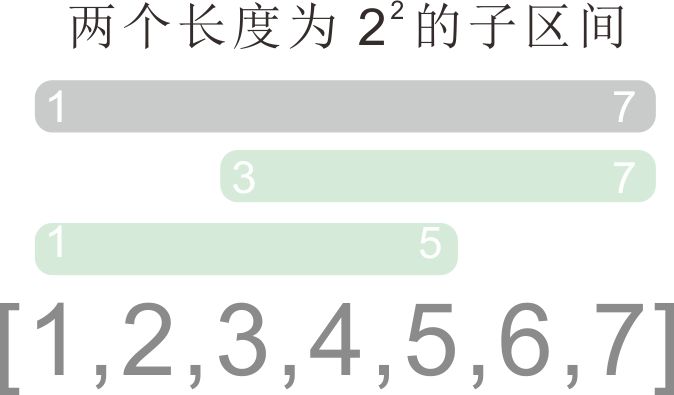ST表的处理过程：

1. 预处理，建立ST表
1. `d[i][j]`为从`i`开始的，长度为`2^j`的闭区间`[i, i+2^j-1]`的最值结果；
2. 使用动态规划，计算出原区间`[l,r]`的全部`d[i][j]`值；
3. 对于区间`[l,r]`，i的取值范围为`[1,r]`，j的取值范围为`[0, log(r-l+1)]`
2. 查询`[l,r]`区间的最值：
1. `[l,r]`区间分为两个子区间`[l,a]``[b,r]`。为了让子区间最大程度地重叠，子区间长度尽可能靠近`r-l+1`，因此`len = 2^j`的指数`j`选取为`j = ⌊ log(r-l+1) ⌋`;
2. 获取`d[l][j]``d[r-2^j+1][j]`的值，取二者的最值即为结果。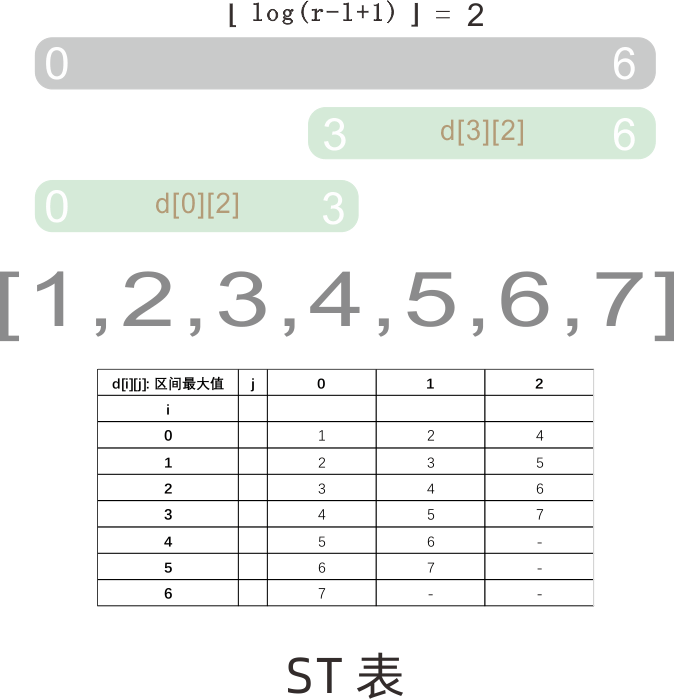### ST表的实现

``````class ST {
constructor(arr = []) {
let JMAX = Math.floor(Math.log2(arr.length)) + 1;
let d = new Array(arr.length).fill(0).map(i => new Array(JMAX).fill(0));
arr.forEach((i, idx) => {
d[idx] = i;
});
for (let j = 1; j <= JMAX; j++) {
for (let i = 0; i + (1 << j) - 1 < arr.length; i++) {
// [i, i+2^j-1] --> [i, i+2^(j-1)-1] + [i+2^(j-1), i+2^j-1]
// d[i][j] --> d[i][j-1] + d[i+2^(j-1)][j-1];
// 动态规划：[i,j]用到j-1列的数据，因此计算顺序是先列后行。
d[i][j] = Math.max(d[i][j - 1], d[i + (1 << (j - 1))][j - 1]); // 这里执行对应的可重复贡献逻辑: max, min, gcd...
}
}
this.d = d;
}
query(l, r) {
let j = Math.floor(Math.log2(r - l + 1));
return Math.max(this.d[l][j], this.d[r - (1 << j) + 1][j]);
}
}
``````

# 数组

## 差分数组

### 定义和适用情况

1. `diff = 0;`
2. `diff[i] = arr[i] - arr[i-1]; (i > 0)`

### 差分数组的性质

• 统一增大n：`diff[a]增大n，diff[b+1]减小n`，其他不变；
• 统一减小n: `diff[a]减小n，diff[b+1]增大n`，其他不变；

### 差分数组的好处

1893.区间是否被全覆盖

★ 1674.使数组互补的最少操作次数

## 子数组

1. 枚举子数组左、右边界：比如统计以某个字符作为结尾的子数组数目，我们枚举找到这个字符的位置，以它为结尾的子数组数目，等于它前面元素的数目`n+1`
2. 枚举子数组中某个特殊元素位置，可以依此将子数组分为左右两部分：比如约定了子数组的最大值、最小值区间范围时，我们直接枚举以某个元素为最大、最小值的子区间数目。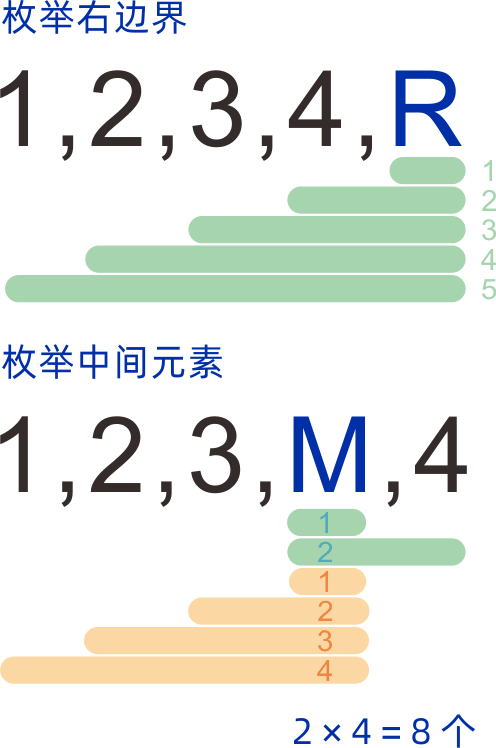#### 最值在某一区间范围`[l,r]`的子数组个数

1. 枚举哪个位置？如果我们选择从左到右遍历原数组，因为我们已遍历的区间在当前位置`i`的左侧，要实现`O(n)`的算法，只能认为我们当前枚举的位置是区间的右边界
2. **如何判断符合要求子区间的数目，以及取舍？
1. 要实现`O(n)`的算法，我们每次只能考虑当前正在遍历的这个元素`arr[i]`，利用它的值来判断以`arr[i]`为右边界的一系列区间的保留舍弃**，因此要设定一个对当前元素的判定条件；
2. 已经遍历的部分，我们记录符合条件的元素出现的长度`len`，以当前位置`arr[i]`为结尾的符合要求子数组个数，就等于`len+1`。（见上节图）；
3. 对于取区间最大值的问题，符合要求的元素为`arr[i] <= MAX`，我们必须保证计入`len`的元素都满足这一条件，因此我们将原区间`[l,r]`划分为`[~,l-1]``[~,r]`两个向左的区间差；
4. 对于取区间最小值的问题，符合要求的元素为`arr[i] >= MIN`，我们必须保证计入`len`的元素都满足这一条件，因此我们将原区间`[l,r]`划分为`[l,~]``[r+1,~]`两个向左的区间差；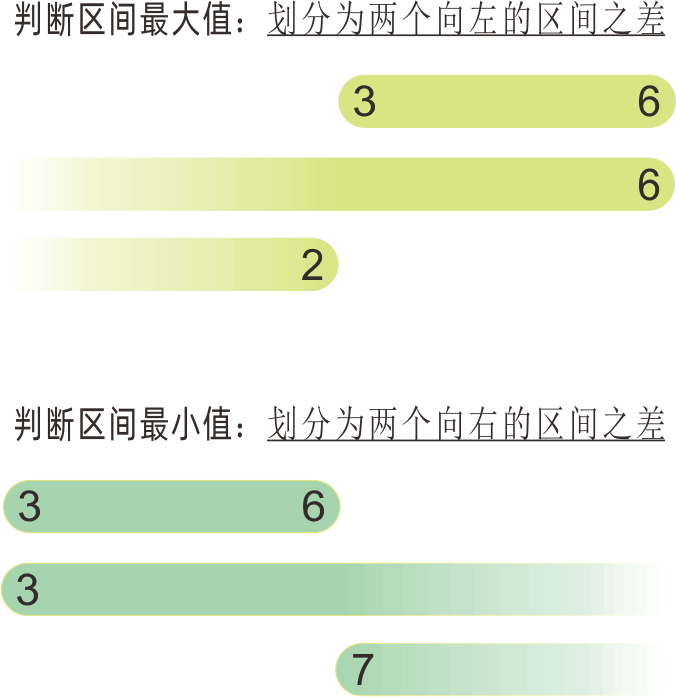``````// 求子数组最小值满足[l,r]的个数：
function minValueBetweenCnt(arr, l ,r) {
// [1,3,2,4,5,10,6,3,1,5,4,9,12,1]
// [3,6]
// min >= 3 && min <= 6
// (min >= 3) - (min >= 7) 区间取最小值，划分为两个向右的区间差
function cntMinValueLargerThan(e) {
let cur = 0;
let res = 0;
for (let i of arr) {
// 枚举子数组右边界位置
if (i >= e) cur += 1; // 当满足要求，连续出现的符合要求元素数 +1
else cur = 0;         // 不满足要求，连续出现的符合要求元素数 归0
res += cur;
}
return res;
}
return cntMinValueLargerThan(l) - cntMinValueLargerThan(r+1);
}

// 求子数组最大值满足[l,r]的个数：(原理相同)
function maxValueBetween(arr, l, r) {
// [l,r]
// [~,r] - [~,l-1]
function cntMaxValueSmallerThan(e) {
let res = 0;
let cur = 0;
for (let i of arr) {
if (i > e) cur = 0;
else cur += 1;
res += cur;
}
return res;
}
return cntMaxValueSmallerThan(r) - cntMaxValueSmallerThan(l-1);
}
``````

### 动态前缀和：树状数组

#### 树状数组（Binary Indexed Array）

315. 计算右侧小于当前元素的个数

2179. 统计数组中好三元组数目

#### 树状数组的结构

1. 树状数组的索引从`1`开始；
2. 树状数组的每一个位置，都保存着原数组`arr`某一区间的加和；
3. `bit[i]`具体保存的是哪一个区间的信息，与索引`i`有关；
4. 从索引`i`二进制表示最末位开始，一直向前直到找到第一个`1`位，区间所表示的二进制数`len = lowbit(i)`，就是`bit[i]`所表示的向前区间长度（包含当前位置）;
5. 某一位置`i`所表示区间的前一个区间元素位置：`i - lowbit(i)`
6. 某一位置`i`所表示区间的上层覆盖区间的元素位置：`i + lowbit(i)`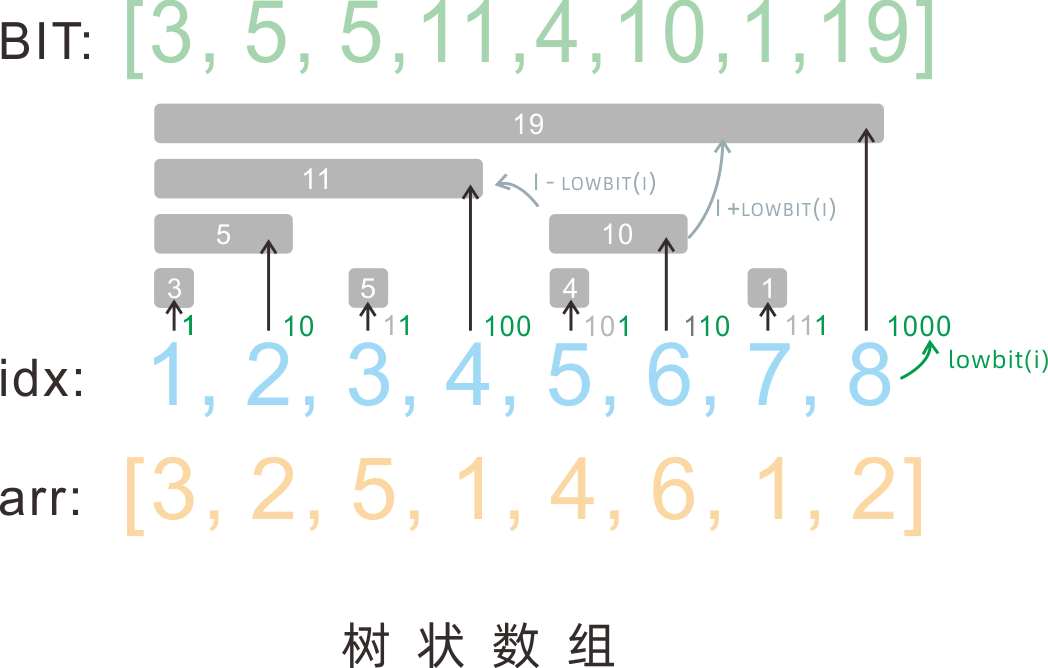#### 树状数组的功能

1. `[1, i]`区间前缀和的查询`query(i)`
2. 原数组`i`位置数据的更新`update(i, diff)`；（diff为更新的增量）
3. `lowbit(i)`，计算`i`位置的区间长度`len`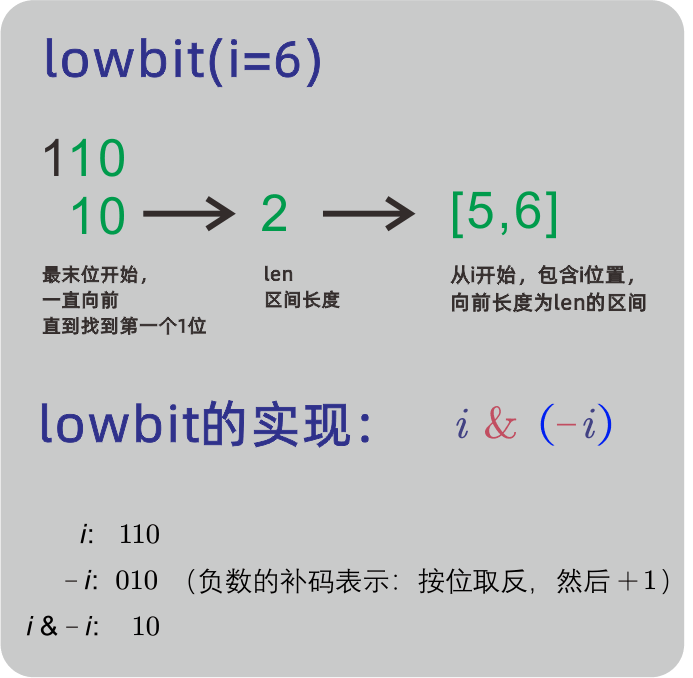#### 树状数组的实现

``````// Mars 2022.02
class BIT {
constructor(arr = []){
// arr[i] -> bit[i+1]
this.bit = new Array(arr.length+1).fill(0);
arr.forEach((i,idx) => this.update(idx, i));
}
lowBit(i) {
return i & (-i);
}
update(i, diff) {
// update arr[i] with delta <diff>.
let c = i + 1;
while (c < this.bit.length) {
// 不断向上寻找覆盖区间，并更新；
this.bit[c] += diff;
c += this.lowBit(c);
}
}
query(i) {
// query prefix sum of [0, i]
if (i >= this.bit.length-1) {
console.warn(`index exceed the limit.`);
return;
}
let c = i + 1;
let r = 0;
while (c > 0) {
// 不断向前寻找区间，并加和；
r += this.bit[c];
c -= this.lowBit(c);
}
return r;
}
}
``````

### 线段树

#### 线段树的结构

1. 线段树是二叉树形式的数据结构，可以用Node数组形式表示；
2. 线段树的每一个节点，代表原数组一个区间的信息。例如：节点A可以表示`[0,3]`区间内，原数组的元素加和结果；
3. 线段树节点的子节点，记录父节点区间，从中间点拆分而成的子区间信息，中间点属于左子节点还是右子节点均可，可以自行约定。

例如：节点A表示`[0,3]`区间，我们约定取中间点为`m = Math.floor((l+r)/2)`，且左子节点保存`[left, m]`的区间，右子节点保存`[m+1, right]`区间，那么A的左子节点保存`[0,1]`区间信息，右子节点保存`[2,3]`区间信息。

4. 线段树的叶子节点，就是区间长度为1的结果，也就是保存了原数组的各个元素值。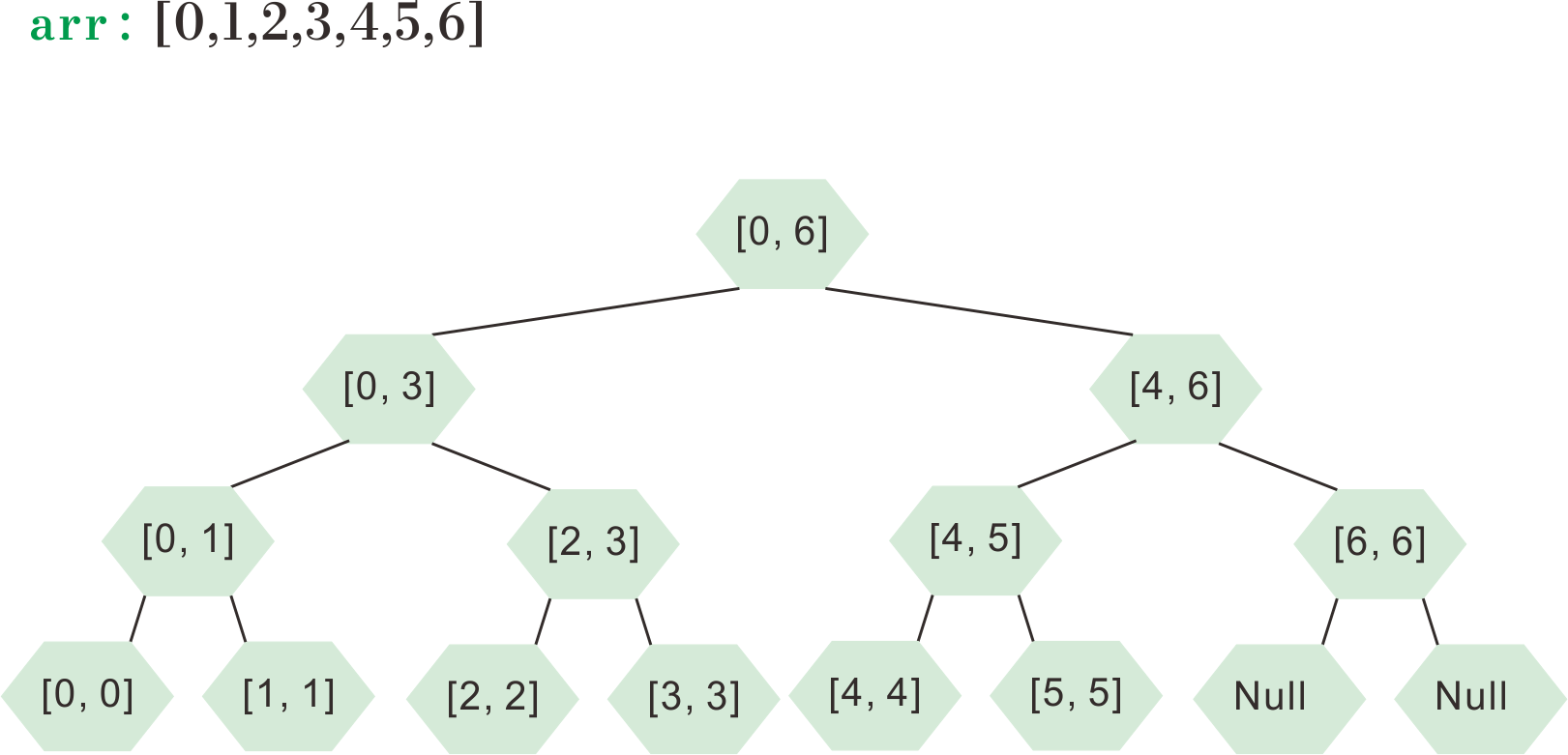``````    constructor(arr = []) {
this.arr = arr;
this.tree = new Array(4 * this.arr.length).fill(null);
this.build();
}
``````

#### 线段树的生成 build

1. 线段树需要的数组空间不好计算，根据渐进公式可以设其长度为`4 * n`(n为原数组长度);
2. 与建堆一样，`i`的左子节点索引为`2i+1`，右子节点为`2i+2`
3. 递归函数需要记录的参数：当前树节点的索引`idx`，当前节点代表的区间左边界`left`，右边界`right`;
4. 递归出口条件：
1. `left === right`: 叶子节点，返回`arr[left]`
2. `left > right`: 空节点，返回null。
5. 函数体内，将区间按约定的中点分隔方式，分隔并递归调用即可。
``````    build(idx = 0, start = 0, end = this.arr.length - 1) {
if (start === end) {
this.tree[idx] = arr[start];
return;
}
let leftIdx = 2 * idx + 1;
let rightIdx = 2 * idx + 2;
let m = Math.floor((start + end) / 2);
// [start, m] [m+1, end]
this.build(leftIdx, start, m);
this.build(rightIdx, m + 1, end);
this.tree[idx] = this.tree[leftIdx] + this.tree[rightIdx];
}
``````

#### 线段树的更新 update

1. 判断更新前后的值是否相同，相同则无需更新，直接结束；
2. 计算要更新的差值，`delta = val - arr[i]`
3. 递归遍历线段树，判断更新的位置`i`是否位于`[left, right]`区间内，是则在当前节点的值上加上差值`delta`，并对子区间递归调用。不在区间内则直接返回；
``````    update(arrIdx, val) {
if (val === this.arr[arrIdx]) return;
let delta = val - this.arr[arrIdx];
this.arr[arrIdx] = val; // refresh arr self
// refresh tree
this._treeUpdate(arrIdx, delta);
}
_treeUpdate(arrIdx, delta, idx = 0, start = 0, end = this.arr.length - 1) {
if (arrIdx < start || arrIdx > end) return;
if (start === end) {
this.tree[idx] += delta;
return;
}
this.tree[idx] += delta;
let leftIdx = 2 * idx + 1;
let rightIdx = 2 * idx + 2;
let m = Math.floor((start + end) / 2);
this._treeUpdate(arrIdx, delta, leftIdx, start, m);
this._treeUpdate(arrIdx, delta, rightIdx, m + 1, end);
}
``````

#### 线段树的查询 query

1. 分治思想，递归将原数组拆分，直到区间两端节点与线段树某一节点的两个端点相同，将线段树中结果加和计入结果中；
2. 返回加和结果。

1. `m`位于查询区间左侧，只需要对右子节点进行递归查询，且区间不用分割；
2. `m`位于查询区间右侧，只需要对左子节点进行递归查询，且区间不用分割；
3. `m`位于查询区间内部，需要将区间分割成`[l, m]``[m+1, r]`，对左右子节点分别进行递归查询、求和。
``````    query(start = 0, end = this.arr.length - 1) {
if (start > end) {
console.warn(`Start must be smaller than or equal to End.`);
return null;
}
return this._query(start, end);
}
_query(qStart, qEnd, idx = 0, start = 0, end = this.arr.length - 1, res = ) {
if (qStart === start && qEnd === end) {
res += this.tree[idx];
return res;
}
let leftIdx = 2 * idx + 1;
let rightIdx = 2 * idx + 2;
let m = Math.floor((start + end) / 2); // [start, m] [m+1, end]
if (m >= qEnd) {
this._query(qStart, qEnd, leftIdx, start, m, res);
} else if (m+1 <= qStart) {
this._query(qStart, qEnd, rightIdx, m+1, end, res);
} else {
this._query(qStart, m, leftIdx, start, m, res);
this._query(m+1, qEnd, rightIdx, m+1, end, res);
}
return res;
}
``````

1146. 快照数组

# 树：二叉树、BST、Trie

## 二叉树 Binary Tree

### 二叉树的特点

• 除了根节点外，它的每个节点入度都是1
• 除了叶子节点外，它的每个节点出度都为2
• 全部节点的入度加和，等于出度加和

### 二叉树的前、中、后序遍历

• 使用一个栈stk来保存遍历的路径，使用Res数组记录结果；
• 因为栈的入栈与出栈顺序相反，所以入栈顺序需要与前、中、后序遍历的顺序相反（前序： 中→左→右，入栈： 右→左→中）；
• 只有在中间元素出栈的时候才记录结果到res数组；
• 在中间元素后面添加一个null元素，作为它出现在中间位且没被res数组记录的标志，一旦在出栈过程中出现null，说明此时应该向res添加记录下一个元素；
• 直到栈stk为空，结束迭代。

``````// 后序遍历：

// 遍历：左右中
// 入栈：中右左
function postOrderIterate(root){
let stk = [root];
let res = [];
while(stk.length !== 0){
let cur = stk.pop();
if(cur === null){
res.push(stk.pop().value);
continue;
}
stk.push(cur, null); // 中 （记录标志为null）
if(cur.right) stk.push(cur.right); // 右
if(cur.left) stk.push(cur.left);   // 左
}
return res;
}
``````

### 二叉树的序列化与反序列化

#### 二叉树该如何序列化

• 如果树中不存在重复的元素，那么知道了二叉树的三种遍历结果中的任意两种，即可还原出原有的树结构。
• 如果存在重复元素，则需要对二叉树的空节点也进行输出，用一个特殊符号表示（将二叉树转化为扩充二叉树），然后进行先序或后序遍历（中序无法确定根节点位置，故不行）。

#### 二叉树的反序列化

`'1234###5###'`为例：

1. 先序遍历结果以`中-左-右`顺序出现，所以最左侧的值一定是当前根节点的值；
2. 先序遍历会沿着一条路径一直向左探索，直到遇见叶子节点；
3. 我们用一个栈`stk`保存路径，每次从序列拿到新的节点值`val`，我们根据`val`的值新建一个节点`Node`（可能是空节点null）：
1. 如果栈顶节点`top`的左子节点为空，则将`val`填充到`top`的左子节点；
2. 如果栈顶节点`top`的左子节点不为空，则将`val`填充到`top`的右子节点；
4. 如果当前值`val`不是代表空节点的占位符`#`，则将新插入的节点入栈，记录下来；
5. 循环判断栈顶元素的左右子节点是否都被填充过，如果是，则对栈顶元素进行出栈，以保证栈顶是下一个待填充的节点；
6. 最后返回根节点即可。
``````function _buildFromPreorderSerialzation(str) {
// Mars 2022.02
if (str.length === 1 && str === '#') return null;
str = str.split('');
let res = new Node(+str, '\$', '\$');
let stk = [res];
for (let i = 1; i < str.length; i++) {
let cur = str[i] === '#' ? null : new Node(+str[i], '\$', '\$');
if (stk[stk.length - 1].left === '\$') stk[stk.length - 1].left = cur;
else stk[stk.length - 1].right = cur;
if (cur !== null) stk.push(cur);
while (stk.length > 0 && stk[stk.length - 1].right !== '\$' && stk[stk.length - 1].left !== '\$') stk.pop();
}
return res;
}
``````

## 字典树 Trie

• 构建、插入： O(n)
• 查询： O(n)

• 构建、插入： O(n)
• 查询： O(1)

### 构建字典树：前缀、后缀1. 初始化：设置根节点为一个空哈希表(Map或空对象)，也可以在内部设置各种结尾的标志符；
2. 插入操作：从插入字符串的0位置（前缀）或末尾位置（后缀）开始，向后（前）遍历，设置初始哈希表cur为根节点，对于每一位的字符letter，执行如下操作：
• ① 在当前哈希表中查找letter键名，如果不存在，则在cur中为letter创建一个新哈希表；
• ② 将cur指向letter对应的哈希表，在letter哈希表设置对应的结束标志为true（如果构建前缀树，每步都设置前缀结束标志为true，最后词尾设置词汇结束标志true）；
• ③ 继续遍历，直到字符串尽头，完成插入；
3. 查询操作：从插入字符串的0位置（前缀）或末尾位置（后缀）开始，向后（前）遍历，设置初始哈希表cur为根节点，对于被查询字符串query每一位的字符letter，执行如下操作：
• ① 在当前哈希表中查找letter键名，如果不存在，则说明没有此查询字符串，返回false结束；
• ② 将cur指向letter对应的哈希表，继续查询；
• ③ query全部查询完毕后，判断此时cur中各结束标志是否为true（比如查询前缀query，那么此时前缀结束标志应该是True），如果为true则返回ture，否则返回false。

# 并查集

## 应用

1. 判断一个图中连通分量的个数

## 基本思路

1. 一个集合用一个代表元素来表示，称为代表元。判断两个集合是否相同，被简化为判断两个集合的代表元是否相同；
2. 一个集合被组织成一个树形结构，代表元是这个树的根元素；
3. 集合中的每个元素`x`，具有根据`x`本身访问它父元素的途径`parent(x)`
4. 根元素的`parent`，指向它自己，也就是`parent(x) = x`
5. 查找一个元素`x`的代表元，只需要不断迭代查询元素的`parent(x)`，即可最终找到集合的根元素；

## 并查集的方法

1. 初始化：每个元素的父节点各自指向自身，因为它们独立组成集合，还没有合并；
2. 查询：两个元素是否在同一集合中；
3. 合并：将两个集合合并成一个；
4. 获取集合中子集合个数：获取当前并查集中子集合的个数。

## 并查集的优化

### 并查集的实现

``````// HashMap Implementation
class BingChaJi {
constructor(vals = []) {
this.map = new Map();
for (let v of vals) {
this.map.set(v, {
rank: 1,
parent: v,
});
}
}

findParent(v) {
let m = this.map;
let p = m.get(v)['parent'];
if (p === v) return v;
else {
let root = this.findParent(p);
this.map.get(v).parent = root;
console.log(`\${v}'s parent is set to \${root}`);
return root;
}
}

isSameSet(v1, v2) {
let root1 = this.findParent(v1);
let root2 = this.findParent(v2);
return root1 === root2;
}

merge(v1, v2) {
let root1 = this.findParent(v1);
let root2 = this.findParent(v2);
if (root1 === root2) return;
let r1 = this.map.get(root1).rank;
let r2 = this.map.get(root2).rank;
if (r1 < r2) {
this.map.get(root1).parent = root2;
console.log(`root is \${root2}`);
} else {
this.map.get(root2).parent = root1;
console.log(`root is \${root1}`);
}
if (r1 === r2) {
this.map.get(root1).rank += 1;
console.log(`rank is set to \${this.map.get(root1).rank}`);
}
}
}
``````

# 图

## DFS深度优先搜索

### 记忆化DFS搜索

638. 大礼包

1. 先对原大礼包数组进行筛选，不划算的礼包中某一物品数量多于所需数量的，都要筛除；
2. 搜索从当前需求`need = needs`开始，对于每一个`need`数组，它都对应着一个`最低购买价格`，我们把求出这个最低价格的函数叫做`getMinPrice()`，则对于需求need的最低价格为`min = getMinPrice(need)`
3. 下面是`getMinPrice()`函数的实现：
1. 最坏情况是，我们的需求，一个大礼包也买不了，这时需要原价买入所有的物品，假设此时价格为`originalPrice`
2. 对于每一个大礼包，我们现在已知买入它们都是划算的（因为筛选过，但它们划算程度不同）。我们要找到所有大礼包中，买入后能满足当前需求，且总价格最低的；
3. 对于每一个大礼包，如果礼包中各物品的数量，都≤当前需求物品数量，则大礼包可以买入。反之，则不能买入。
4. 我们遍历所有大礼包，对于一个大礼包`s`，它内部物品数目列表为`sn`，如果它可以买入，那么它买入后，下次我们的需求就变成了`need - sn`（数组各位分别减去对应物品数目）。那么它买入后需求的最低购入价格就是`getMinPrice([need - sn])`，因此，买了这个礼包后，我们的购入价格是`price_need = getMinPrice([need - sn]) + price_s`
5. 遍历所有大礼包，对每一个能买入的礼包`s`，都计算`price_need = getMinPrice([need - sn]) + price_s`，则达成当前需求need的最低费用是: 原价购买价格`originalPrice`与所有`price_need `中的最小值；
4. 每次找到当前need的最低购入价格，就把它放在备忘录`map`中，键名是当前need形成的字符串`need.join('-')`，键值是价格；
5. 如果当前`need`的最低购入价格在备忘录中存在，则直接返回，省去计算步骤；
6. 要找的结果是最后备忘录中初始需求`needs`对应的价格。

## BFS广度优先搜索

### JS广度优先搜索的优化

``````// template
let q = [init];
while (q.length) {
let next = []; // 新next数组
while (q.length) {
let cur = q.pop(); // 从原队列pop()
let n = cur.next;  // 找到下一层结点
next.push(n);  // push到next
}
q = next; // 用next代替q
}
``````

## 拓扑排序

OI Wiki拓扑排序

### 实现

• Kahn算法：从入度为0的节点开始，正序记录（在入度为0的时候记录数据）；
• DFS：在出度为0的时候记录数据，记录顺序为逆序。

#### Kahn 算法

Kahn算法基本过程：

1. 遍历有向无环图，记录每个节点的下一相邻节点集合，以及节点的入度（进入该节点的边数）
2. 遍历所有节点，找到初始入度为0的节点，组成初始队列`q`
3. 每次从队列`q`中出列一个节点`n`，然后执行如下操作：
1. `n`添加到结果数组`res`尾部;
2. 找到`n`全部的相邻下一节点`t`，将`t`的入度`-1`（等同于将`n`从图中删除）；
3. 如果`t`的入度此时为0，则将`t`加入队列`q`尾部；
4. 直到队列`q`为空，停止遍历，`res`为拓扑排序结果。

``````function kahnSort(g) {
let entryNum = new Map();
let tos = new Map();
for (let [f, t] of g) {
if (!entryNum.has(f)) entryNum.set(f, 0);
if (!entryNum.has(t)) entryNum.set(t, 0);
entryNum.set(t, entryNum.get(t) + 1);
if (!tos.has(f)) tos.set(f, new Set());
}
let q = [];
let res = [];
for (let [k,v] of entryNum.entries()) {
if (v === 0) q.push(k);
}
while (q.length) {
let i = q.shift();
res.push(i);
if (tos.has(i)) {
for (let t of tos.get(i)) {
entryNum.set(t, entryNum.get(t)-1);
if (entryNum.get(t) === 0) q.push(t);
}
}
}
return res;
}
``````

#### DFS 实现拓扑排序

DFS实现拓扑排序基本过程：

1. 用一个数组`visited`，标记节点是否被记录；
2. 遍历图，记录每一节点`n`的下一相邻节点集合，集合的元素数目就是`n`出度
3. 从任意节点开始，遍历全部节点，对任一节点`n`，如果其未被遍历:
1. 如果出度为0，则push`n`到结果数组`res`;
2. 如果出度不为0，则递归对每一相邻下一节点`t`执行`dfs(t)`
3. 记录`visited[n]`为true；
4. 执行完毕，`res`为倒序的拓扑遍历结果。
``````function dfsSort(g) {
let m = new Map();  // 记录下一邻接节点集，以及出度
let nodes = new Set(); // 记录全部节点集合
for (let [f,t] of g) {
if (!m.has(f)) m.set(f, new Set());
}
let res = [];
let visited = new Set(); // 已访问节点集合

function dfs(n) {
if (!visited.has(n)) {
if (!m.has(n)) {
res.push(n);
return;
}
for (let c of m.get(n)) {
dfs(c);
}
res.push(n);
}
}

for (let i of nodes) {
dfs(i);
}

return res.reverse();
}
``````

## 最小生成树

### 最小生成树：Kruskal算法

Kruskal算法属于贪心算法。它的流程如下：

1. 用并查集维护节点的连通性关系；
2. 维护已添加的边的权重加和`sum`，以及添加的边数`num`
3. 从所有边中，选出权值最小的边`a -- b`，查看`a``b`是否在同一个连通分量中：
1. 如果不在，则将这个边的权值计入结果`sum`，并将边数`num += 1`;
2. 如果在同一个连通分量，则跳过，寻找下一个权值最小边长；
4. 直到添加了`n-1`条边，结束返回。# 动态规划（Dynamic Programming）

1. 区分操作和状态：操作是对状态进行转移的动作。首先要区分题目中哪些是可进行的操作，哪些是问题的状态；
2. 定义状态：找到问题所描述的解的状态，从而确定定义一个什么样的状态，可以由状态转移逐步推导出问题的解；
3. 寻找状态变量：找寻合适的参数变量集合，使得一组确定的参数变量，可以确定问题唯一的状态；
4. 给定初始状态或状态的边界： 无法再分割的最小状态，往往对应着单一且显而易见的结果，直接将结果赋值，然后从这些边界状态开始递推；
5. 找到状态转移方式： 找到由旧状态递推新状态的方法。

## 背包（零钱）问题

• 0-1背包问题： 背包内的东西只能取一次；
• 完全背包问题： 背包内的东西是无限的。

0-1背包问题:

### 0-1背包问题

`dp[r][c]`的含义是： 背包剩余容量为`c`的情况下，仅由`[0,r]`中的物品组成，能装下的最大价值。

• 选择装下这个物品r：假设物品r本身重量为wr，价值为vr，那么背包剩余容量为c-wr，物品r已经装入，只能从`[0,r-1]`物品区间继续装入，此时能装下的最大价值为`dp[r-1][c-wr]`。 因此这种情况的最大价值为`vr+dp[r-1][c-wr]`
• 放弃装这个物品r：最大价值与r-1个物品时一样。为`dp[r-1][c]`

### 完全背包问题

0-1背包问题中，每个物品只能选择一次。而完全背包问题中，每个物品的数目是无限的，可以重复选择。

• ① 如果先遍历amount，后遍历notes，则意味着：对于每个目标值，都直接穷举出所有当前可能的钞票组合，之后再进入下一个目标值进行更新。

• ② 如果先遍历notes，后遍历amount，则意味着：对于每个钞票note，先计算并更新单独由这个note组成各个目标值的方法数，然后再进入下一个面额note，再更新这个新面额和之前所有面额组成的方法数，保证了每个方案中的钱的排布顺序，是`notes`的顺序。

## 股票买卖

1. 一天可以有三种选择：买入、卖出、不操作；
2. 只有卖出才能获得收益；
3. 卖出必须先买入；
4. 如果不操作，则总收益和之前一天的收益相同。

• 如果卖出， 则之前必定有一天进行了买入。此次交易赚取收益为`price[sell]-price[buy]`
• 如果不卖出，则总收益与前一天收益相同；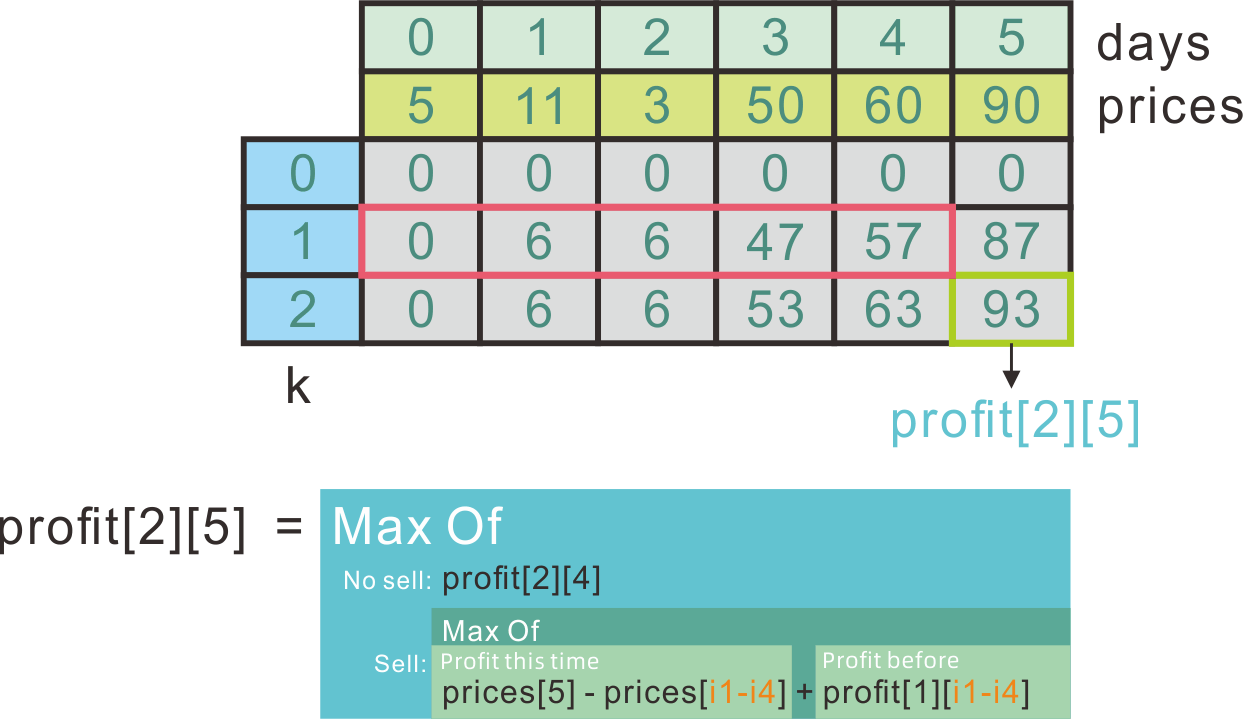``````function maxProfitWithKTransactions(prices, k) {

if(prices.length < 2) return 0;

let dp = new Array(2);
dp = new Array(prices.length) .fill(0);
dp = new Array(prices.length);
let trans = 1;
while(trans <= k){
for(let i=0; i<prices.length; i++){
if(i === 0) dp[i] = 0;
else {
let notSellMaxProfit = dp[i-1];
let sellMaxProfit = -Infinity;
}
dp[i] = Math.max(notSellMaxProfit, sellMaxProfit);
}
}
dp = dp;
dp = new Array(prices.length);
trans++;
}
return dp[prices.length-1];
}
``````

## 三个无重叠子数组最大和

1. 先遍历数组，用一个map记录以i位置为结束位置的长度为k的子数组的加和，i<k-1的值都为0，因为元素数目不够。（map.get(i)是`[i-k+1, i]`区间的加和）；
2. 使用一个二维dp数组保存计算结果。
• dp的行n取值从0到3，代表可以选取的不相交子数组个数；
• dp的列i取值从0到nums.length-1，代表结束位置；
• 因此，`dp[n][i]`代表可以取n个子数组时，`[0,i]`区间内可以取到的n个子数组的最大和。
3. 当n=0时，`dp[n][i]`全部为0；其他情况下，当进行`dp[n][i]`的取值时，分为以下两种情况：
• 不使用当前位置i构成的子数组map.get(i)：`dp[n][i] = dp[n][i-1]`
• 使用当前位置i构成的子数组map.get(i): 则子数组`[i-k+1,i]`一定被使用了，当前dp值应该为 `dp[n][i] = dp[n-1][i-k] + map.get(i)`
4. 当n=3，保留结果中的最大值和它对应的索引值index；
5. 反向查找dp表，获得各子数组起始索引：
• 对于一个值`dp[n][i]`，如果它使用了i位置的子数组，那么`dp[n][i]`一定大于`dp[n][i-1]`
• 从n=3开始找，一直到n=1，保存结果值返回即可。
``````var maxSumOfThreeSubarrays = function(nums, k) {
let map = new Map();
let first = 0;
for(let i=0; i<k; i++){
first += nums[i];
map.set(i,0);
}
map.set(k-1, first);
for(let i=k; i<nums.length; i++){
let cur = nums[i];
let prev = nums[i-k];
let sum = map.get(i-1) + cur - prev;
map.set(i, sum);
}

let dp = new Array(4).fill(0).map(i => new Array(nums.length).fill(0));
let max = -Infinity;
let index = 0;
for(let n=1; n<4; n++){
for(let i=0; i<nums.length; i++){
let notUsed = i-1 >= 0 ? dp[n][i-1] : 0;
let used = i-k >= 0 ? map.get(i) + dp[n-1][i-k] : map.get(i);
dp[n][i] = Math.max(notUsed, used);
if(n === 3 && dp[n][i] > max){
max = dp[n][i];
index = i;
}
}
}

function findIndex(i){
let n = 3;
let res = [0,0,0];
while(n > 0){
while(i >= 0 && dp[n][i] === dp[n][i-1]){
i--;
}
res[n-1] = i-k+1;
i = i-k;
n--;
}
return res;
}

return findIndex(index);
};
``````

## 最长回文子序列

Leetcode516: 最长回文子序列

1. 回文序列首尾元素一定相同；
2. 设置一个二维dp数组，`dp[i][j]`代表从`[i,j]`区间的最长子序列长度；
3. `dp[i][j]`递推根据首尾元素是否相同判断：
• 如果首尾元素相同，则`dp[i][j] = dp[i+1][j-1] + 2`;
• 如果首尾元素不同，则它们必定不能同时作为最长子序列的首尾，`dp[i][j] = max (dp[i+1][j], dp[i][j-1])`
4. 需要注意dp遍历顺序问题，如果j从0到字符串末尾，那么i需要从j-1递减到0，这样才能正确利用之前的计算结果。

## 从1到n的BST的种类数

1. 对于一个数字`n`，那么从1到n，每一个数都可以选取作为根节点；
2. 根据BST的性质，如果选择了i作为根节点，那么：
• `[1,i-1]`的元素都在左子树；
• `[i+1,n]`的元素都在右子树。
3. 根据BST的规则，`[1,n]`的BST种类和`[1+K,n+K]`的BST种类相同；
4. `dp[i]``[1,i]`区间可构建的BST种类数，那么：
• `[1,i-1]`的元素都在左子树，构建的BST种类数为`dp[i-1]`
• `[i+1,n]`的元素都在右子树，构建的BST种类数为`dp[n-i]`
5. 对于一个根节点，总种类数为左右子树种类数相乘，则有状态转移方程：`dp[i] = dp[i-1] * dp[n-i]`
6. 从1到n遍历`n`，对每一个`n`遍历`i = [1,n]`选取作为根节点，计算其种类数加和做为`dp[n]`
7. 返回`dp[n]`即可。

## 区间DP

1. `dp[l][r]`代表闭区间`[l,r]`范围内的结果；
2. `[l,r]`区间进行某种拆分，常见的是在`[l+1,r-1]`区间枚举切分点`i`，将区间分为`[l,i]``[i,r]`两部分，并对每个切分点结果进行比较取最值，记录到`dp[l][r]`
3. 注意区间DP的顺序问题：因为大区间要用到小区间的信息，所以遍历的时候要先以区间长度`len`为条件（从小到大），再枚举区间的起点`l`（从小到大、从大到小均可），`r`的位置此时可以确定为`l+len`

1039. 多边形三角剖分的最低得分

• 假设`dp[l][r]``[l,r]`区间切分的最小得分，那么整体的最小得分就是`dp[len-1]`
• `[l+1,r-1]`区间内可以枚举切分点，将区间分为`[l,i]``[i,r]`两部分；
• `dp[l][r] = min(dp[l][i] + dp[i][r] + v[l]*v[r]*v[i])`
``````var minScoreTriangulation = function(values) {
// 区间DP
// dp[l][r]: [l,r] 区间的最低分。
// dp[l][r] = min(v[l]*v[r]*v[k] + dp[l][k] + dp[k][r]), k -> [l+1, r-1];
let dp = new Array(values.length).fill(0).map(i => new Array(values.length).fill(Infinity));
for (let len = 1; len <= values.length-1; len += 1) {
for (let l=0; l+len<values.length; l++) {
if (len === 1) {
dp[l][l+len] = 0;
continue;
}
for (let i=l+1; i<=l+len-1; i++) {
dp[l][l+len] = Math.min(dp[l][l+len], values[l]*values[l+len]*values[i] + dp[l][i] + dp[i][l+len]);
}
}
}
return dp[values.length-1];
};
``````

# 分治算法

## 平面最近点对

### 思路

1. 直接暴力求解，问题复杂度为`O(n^2)`，复杂度高的原因是进行了大量的无意义计算（比如相隔非常远的点间距离）；
2. 可以用一条线将平面分割成两部分（假定选择垂直于X轴的竖线），发现问题可以被分为子问题：
1. 左边部分点集的最小距离`l_min`
2. 右边部分点集的最小距离`r_min`
3. 横跨左右点集的最小距离`m_min`
3. 对于任何一个点集的最终结果，都是上述三个子区间各自结果中的最小值`min(l_min, r_min, m_min)`
4. 采用分治算法：
1. 先对点集进行按x坐标从小到大排序；
2. 递归出口：如果区间内的元素小于`3`个，直接进行计算返回；
3. 按上述划分法，对每一个区间`[l,r]`，取中间点`m = Math.floor((l+r)/2);`，分割成左右两部分；
4. 对左右两部分分别递归求最小距离，假设结果为`l_min``r_min`，我们取它们二者中的最小值为`delta`
5. `m`位置的左右两侧，只对`± delta`范围内的元素进行求距离比对，找出这个范围内的最小距离`m_min`
6. 返回三者中的最小值。

### 实现

``````function getDist(p1, p2) {
return Math.sqrt((p1 - p2) ** 2 + (p1 - p2) ** 2);
}

function closestPair(points, l = 0, r = points.length - 1) {
if (r - l <= 2) {
if (r - l === 1) return getDist(points[r], points[l]);
let a = getDist(points[l], points[l + 1]);
let b = getDist(points[l + 1], points[r]);
let c = getDist(points[l], points[r]);
return Math.min(a, b, c);
}
let m = Math.floor((l + r) / 2);
let lmin = closestPair(points, l, m);
let rmin = closestPair(points, m+1, r);
let delta = Math.min(lmin, rmin);
// +- delta
let leftSet = new Set();
let rightSet = new Set();
let c = m;
while (c >= 0 && Math.abs(points[c] - points[m]) <= delta) {
c -= 1;
}
c = m + 1;
while (c < points.length && Math.abs(points[c] - points[m]) <= delta) {
c += 1;
}
for (let p1 of leftSet) {
for (let p2 of rightSet) {
lmin = Math.min(lmin, getDist(p1, p2));
}
}
return Math.min(lmin, rmin);
}

// test
let p = [[1,2],[2,1],[3,200]];
p.sort((a,b) => a - b);
console.log(closestPair(p)); // 1.414..
``````

## 为运算表达式设计优先级

241. 为运算表达式设计优先级

• 设置一个dfs(l,r)函数，用来计算原表达式从l到r闭区间内子表达式的计算结果；
• 原表达式可以从任一操作符一分为二，可知左右两侧仍是一个表达式；
• 对两侧表达式重复上述分割步骤，直到两侧为不含操作符的数字；
• 每一个表达式因计算优先级的不同，可能有多种计算结果，因此返回的应该是一个数组，里面保存了所有表达式可能的计算结果；
• 每次用当前运算符，对左右两个表达式的计算结果进行遍历计算，合并结果集，最终返回整个表达式的结果。
``````var diffWaysToCompute = function(expression) {
// 测试字符串是否是纯数字
function isNumber(str) {
return /^\d+\$/i.test(str);
}
// 执行单次计算
function cal(l, r, o) {
switch (o) {
case '+': {
return l+r;
}
case '-': {
return l-r;
}
case '*': {
return l*r;
}
}
}
// 按操作符分隔表达式，取结果集合。
function dfs(l = 0, r = expression.length-1) {
if (isNumber(expression.slice(l,r+1))) {
return [+expression.slice(l,r+1)];
}
let res = [];
for (let i=l; i<=r; i++) {
if (!isNumber(expression[i])) {
let left = dfs(l, i-1);
let right = dfs(i+1, r);
let operater = expression[i];
for (let i of left) {
for (let j of right) {
res.push(cal(i, j, operater));
}
}
}
}
return res;
}

return dfs();
};
``````

# 回溯算法

## 算法基本思路

• 当前路径：是由已经进行过的所有决策组成的。下一步决策依赖于当前走过的决策路径。（比如：有三张牌ABC，第一次选择B，第二次选择C，则当前第三次选择的路径为B-C）；
• 当前决策空间：根据当前路径，本次决策剩余的选择空间。（ABC三张牌，前两次选择BC，则第三次当前选择空间只有一张牌A）；
• 结束条件：决策空间中没有任何选项时，则代表决策树遍历到尽头，需要用结束条件处理这次决策的结果。（比如将当前决策路径B-C-A保存起来）

1. 先判断当前路径是否满足结束条件，满足则执行结束操作；
2. 在当前决策空间B内进行决策，然后在当前路径A中添加当前决策（结果为A1），在当前决策空间B中去掉当前决策（结果为B1）；
3. 以A1为下一决策的路径，以B1为下一决策的决策空间，递归调用自身；
4. 调用完成后，将决策路径B1恢复为B，路径A1也恢复为A。（这一步称为回溯过程）

## 回溯的注意事项

1. 回溯算法复杂度高(`O(n!)`)，一般数据量要小于20；
2. 回溯算法本质上是递归，一般都可以应用记忆化方法，记录计算结果，防止重复计算；
3. 回溯计算过程中，如果找到了想要的结果，要及时提前退出递归，终止后续计算。

## 回溯算法：全排列问题

### 元素互不相同的全排列

1. 当前路径就是已经选择过的字母序列，当前决策空间就是剩余的可被选择的字母集合；
2. 每次决策，在剩余字母中选择一个(比如’a’)，添加到路径中，然后在可选择字母集合中删除掉这个已经选过的字母a；
3. 使用新路径和新决策空间，递归调用执行下一次决策；
4. 结束条件：当可选择字母集合为空，说明排列已经完成，将结果添加到结果集合，然后返回；
5. 递归调用完成后，恢复路径（删掉添加的字母a）和决策空间（恢复字母a）。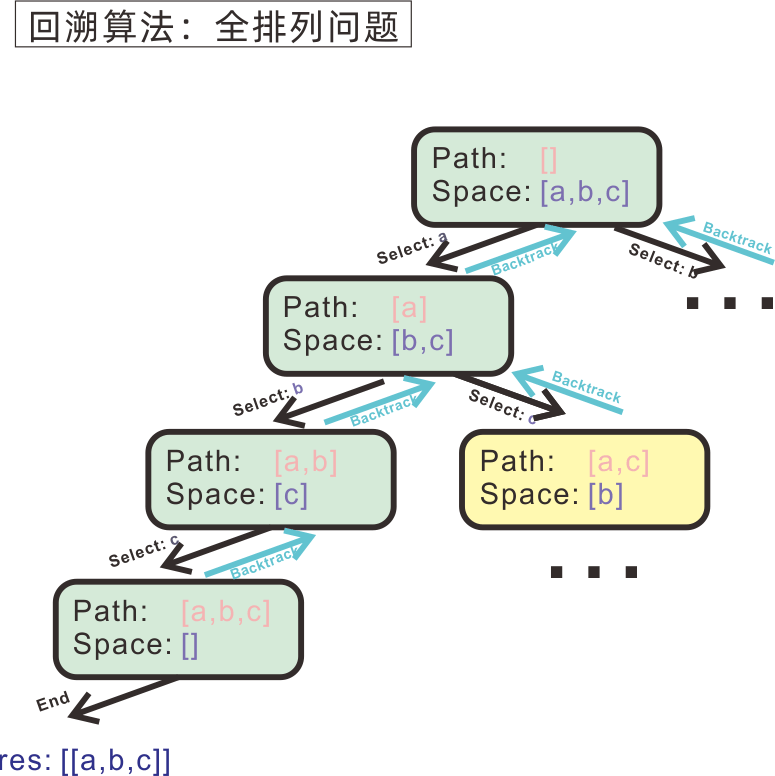``````// 方法1：
function getArrangement(arr, path=[], res=[]){
// arr是当前决策空间，path是当前路径
// 1.判断结束条件：决策空间内无元素可选；
if(arr.length === 0){
res.push(path.slice());
return;
}
for(let i=0; i<arr.length; i++){
// 2.进行决策；
let selected = arr[i];
// 3. 修改决策空间和路径，用来进行下一次决策；
path.push(selected);
arr.splice(i, 1);
// 4. 递归调用，执行下一次决策；
getArrangement(arr, path, res);
// 5. 恢复 决策空间 和 路径。
arr.splice(i, 0, selected);
path.pop();
}
return res;
}

// 方法2： 使用一个used记录选择的路径
function allArrangement2(arr){
let path = [], used = new Array(arr.length).fill(false);
let res = [];

function backtrack(){
if(path.length === arr.length){
res.push(path.slice());
return;
}
for(let i=0; i<arr.length; i++) {
if (!used[i]) {
path.push(arr[i]);
used[i] = true;
backtrack();
used[i] = false;
path.pop();
}
}
}

backtrack();
return res;
}
``````

### 元素存在重复的全排列

``````function backtrack(path=[], space=nums, res=[]){
if(space.length === 0){
res.push(path.slice());
return;
}
// 为每一轮回溯决策过程，设置一个Set，用来保存已经选择过的元素值。
let set = new Set();
for(let i=0; i<space.length; i++){
// 当该决策空间中已经选取过当前元素，直接跳过，防止重复。
if(set.has(space[i])) continue;
else {
let cur = space[i];
path.push(cur);
space.splice(i,1);
backtrack(path, space, res);
space.splice(i,0,cur);
path.pop();
}
}
return res;
}
``````

## 全部子集（组合问题）

1. 设置一个path，代表已经选择的元素路径；
2. 对于每一个path，设置一个start值，代表下次选择的起始位置；（含义是：path条件下，`index < start`的全部元素子集已经处理完毕）
3. 每次从start到数组结尾遍历剩余选择空间，将元素放入path然后记录到结果数组，最后返回结果数组。

``````function allCombination(arr){

function backtrack(path = [], start = 0, res = []){
// 对于每一个path，选择空间是[start, arr.length-1]，[0,start) 全部子集都处理完成，不用考虑。
for(let i=start; i<arr.length; i++){
path.push(arr[i]); // 对于每一个可选择元素，我们可以将其加入组合，也可以选择跳过（不加入）。
res.push(path.slice());
backtrack(path, i+1, res);
path.pop(); // 回溯，当前元素arr[i]被选择的情况已经遍历完成，将其弹出（表示当前元素被跳过），继续下一个元素的决策。
}
return res;
}

return backtrack();
}
``````

### 组合的去重

1. 先将数组排序，这样重复的元素位置会相邻分布；
2. 在回溯寻找组合的过程中，对于某次元素选择`arr[i]`，查看其上一元素`arr[i-1]`是否与它相同，如果相同则跳过。
``````var subsetsWithDup = function(nums) {
nums.sort((a,b) => a-b); // 先排序，让相同元素凑在一起。
function backtrack(path = [], start = 0, res = []){
for(let i=start; i<nums.length; i++){
if (i > start && nums[i] === nums[i-1]) continue; // 这里判断当前元素与前一元素是否相同，相同则跳过。（同一深度，添加相同的元素会造成重复）
path.push(nums[i]);
res.push(path.slice());
backtrack(path, i+1, res);
path.pop();
}
return res;
}
return backtrack();
};
``````

## 回溯算法：括号匹配

``````var generateParenthesis = function(n) {

function backtrack(path=[], leftleft=n, rightleft=n, res=[]){
// 结束条件
if(leftleft === 0 && rightleft === 0){
res.push(path.join(''));
return;
}
// 回溯：选择
if(leftleft > 0){
path.push('(');
backtrack(path, leftleft-1, rightleft, res);
path.pop();
}
// 这里可以保证：右括号只有在有左括号能匹配的情况下，才会被添加。
if(rightleft > leftleft){
path.push(')');
backtrack(path, leftleft, rightleft-1, res);
path.pop();
}

return res;
}

return backtrack();
};
``````

# 递归

## 递归的特点和基本思想

• 设置合理的退出条件；
• 递归深度影响空间复杂度，能用迭代实现尽量不用递归。

## 典型递归问题

1. 控制可用的开始和结束标签数目，当开始标签可用数>0时，向后面添加一个开始标签；
2. 然后检查结束标签可用数，如果发现结束标签可用数大于开始标签可用数，说明有开始标签没有被正确关闭，此时向字符串后添加一个结束标签；
3. 当开始标签和结束标签可用数都为0，则向结果数组中保存结果。
``````function generateDivTags(numberOfTags) {
let res = [];
function getStrings(prefix, openings, closings, result){
if(openings === 0 && closings === 0){
result.push(prefix);
}
if(openings > 0){
let newPrefix = prefix + '<div>';
getStrings(newPrefix, openings-1, closings, result);
}
if(closings > openings){
let newPrefix = prefix + '</div>';
getStrings(newPrefix, openings, closings-1, result);
}
}
getStrings('', numberOfTags, numberOfTags, res);
return res;
}
``````

# 排序算法

1792. 最大平均通过率

## 字典序

1. 从前到后逐位比较，同一位置上字符值更大的，字典序更靠后；
2. 前缀相同的两个字符串，长度更长的，字典序靠后；

• 它可以按照给定的字母顺序，形成一个`n叉字典树`
• 将字符串按字典序排列，等同于将字符串加入这个字典树，然后对这个`n叉树`进行先序遍历

# 搜索算法

## 二分查找

### 二分查找适用情况

• ①顺序存储：数组结构；
• ②有序，可以缩小结果范围。

### 二分查找的关键信息

• 成功应用二分查找，必须要找到一个根据中间点，能对左右两侧区间进行取舍的判据
• 二分查找的最终时间复杂度为`O(log_n)`

### ★二分查找的区间情况和对应的代码

1. `[a,target)``[target,b]`;
2. `[a,target]``(target,b]`

• `≤target`的最后一个元素；
• `<target`的最后一个元素；
• `≥target`的第一个元素；
• `>target`的第一个元素。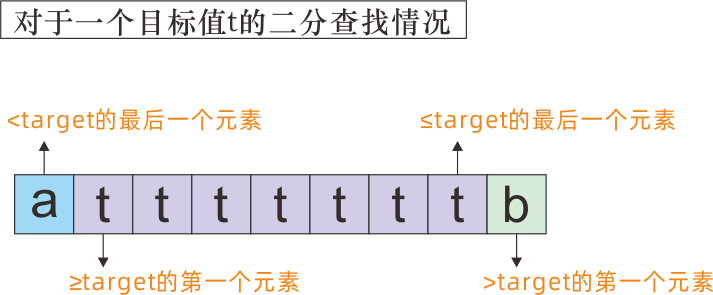• 查找`≤target`的最后一个元素时：
• mid ≤ target: left = mid;
• mid > target: right = mid - 1;
• mid的取整情况：向上取整。
• 查找`<target`的最后一个元素:
• mid < target: left = mid;
• mid ≥ target: right = mid - 1;
• mid的取整情况：向上取整。
• 查找`≥target`的第一个元素:
• mid < target: left = mid + 1;
• mid ≥ target: right = mid;
• mid的取整情况：向下取整。
• 查找`>target`的第一个元素:
• mid ≤ target: left = mid + 1;
• mid > target: right = mid;
• mid的取整情况：向下取整。

1. 先正确切分区间（哪边闭哪边开），然后判断查找的目标区间在哪一侧，目标区间边界在收缩的时候一定是闭的（在左侧：`left = mid` 或 在右侧：`right = mid`）；
2. right只能向左缩小，left只能向右缩小。也就是说，left、right取值只有以下两种情况：
• left = mid, right = mid - 1;
• left = mid+1, right = mid;
3. 向上还是向下取整，取决于哪边是开区间：
• 当存在left = mid + 1，向下取整；
• 当存在right = mid - 1， 向上取整。
4. 二分查找过程中，mid的判断条件，和区间的切分情况一定完全相同。需要做的只是根据区间的切分情况，对mid进行开闭取舍；
5. mid位于目标值target左侧，动左区间。mid位于目标值右侧，动右区间。

``````Math.floor(((2 ** 31) - 1)/2)  // 1073741823
((2 ** 31) - 1) >> 1           // 1073741823
Math.floor(((2 ** 32) - 1)/2)  // 2147483647
((2 ** 32) - 1) >> 1           // -1
``````

### 基本代码实现

``````// 递归实现：升序数组nums中，查找≤target的最后一个元素。
const search = function(nums,target) {
function find(left, right){
if(left < right){
let mid = Math.ceil((left+right)/2);
if(nums[mid] <= target) return find(mid, right);
else return find(left, mid-1);
}
return left;
}
return find(0,nums.length-1);
};

// 迭代实现
var search = function(nums,target) {
let left = 0, right = nums.length-1;
while(left < right){
let mid = Math.ceil((left+right)/2);
if(nums[mid] <= target) left = mid;
else right = mid-1;
}
return left;
};
``````

### 二（多）维空间的二分查找

1. 先确定元素的左右极值：因为为有序排列，极值位于维度增长方向的两个端点；

例如：

对于二维数组`arr`，如果按行按列都是递增的，那么极小值在`arr`，极大值在`arr[arr.length-1][arr.length-1]`，也就是左上角、右下角;

2. 对于一个`k`维有序数组，我们可以在O(nk-1)时间复杂度完成一次查询，方法是：固定其他维度，将多维降为二维，然后找到二维数据某一递增轴的末端点，作为起点，向另一个轴方向进行计数；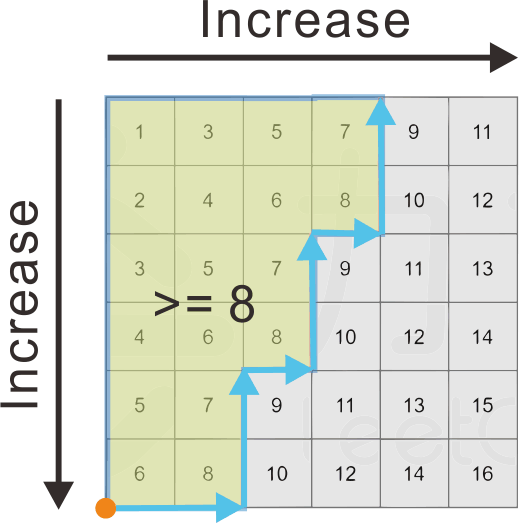3. 然后利用左右极值，进行常规二分查找即可。

# 滑动窗口

— 《挑战程序设计竞赛》

1. 通过左右边界维护一个窗口：`left``right`，初始都在`0`位置;
2. 窗口的有效区间范围是`[left, right]`双闭区间；
3. 右边界是主动前进的，它负责向右扩展窗口探索新区域，每次只前进一步
4. 左边界是被动前进的，因为每次右边界都会前进，窗口内的值可能不再符合要求，需要收缩左边界使得当前窗口内的值继续满足要求，左边界每次可以前进多步
5. 直到右边界到达末尾`length-1`位置，停止搜索。
``````// 滑动窗口的伪代码
let left = 0, right = 0;
let target = 0, res = 0;
while (right < arr.length) {
target += arr[right];
while (/* target do not fit the condition */) {
target -= arr[left]; // decrease length of the window, change the target value.
left += 1;
}
res = target // record the found answer here.
right += 1;
}
return res;
``````

# 枚举

6029. 射箭比赛中的最大得分

## 快慢指针

• 链表寻环： 使用快慢指针，快指针比慢指针每次多走1步，如果有环存在，则快慢指针一定会相遇；
• 寻找链表的中点； 使用快慢指针，慢指针每次走1步，快指针每次走2步，则快指针到达尾部，慢指针正好在链表中间位置；

# 有序集合

## 跳表 SkipList

Redis为什么用跳表而不用平衡树？ - 张铁蕾的文章 - 知乎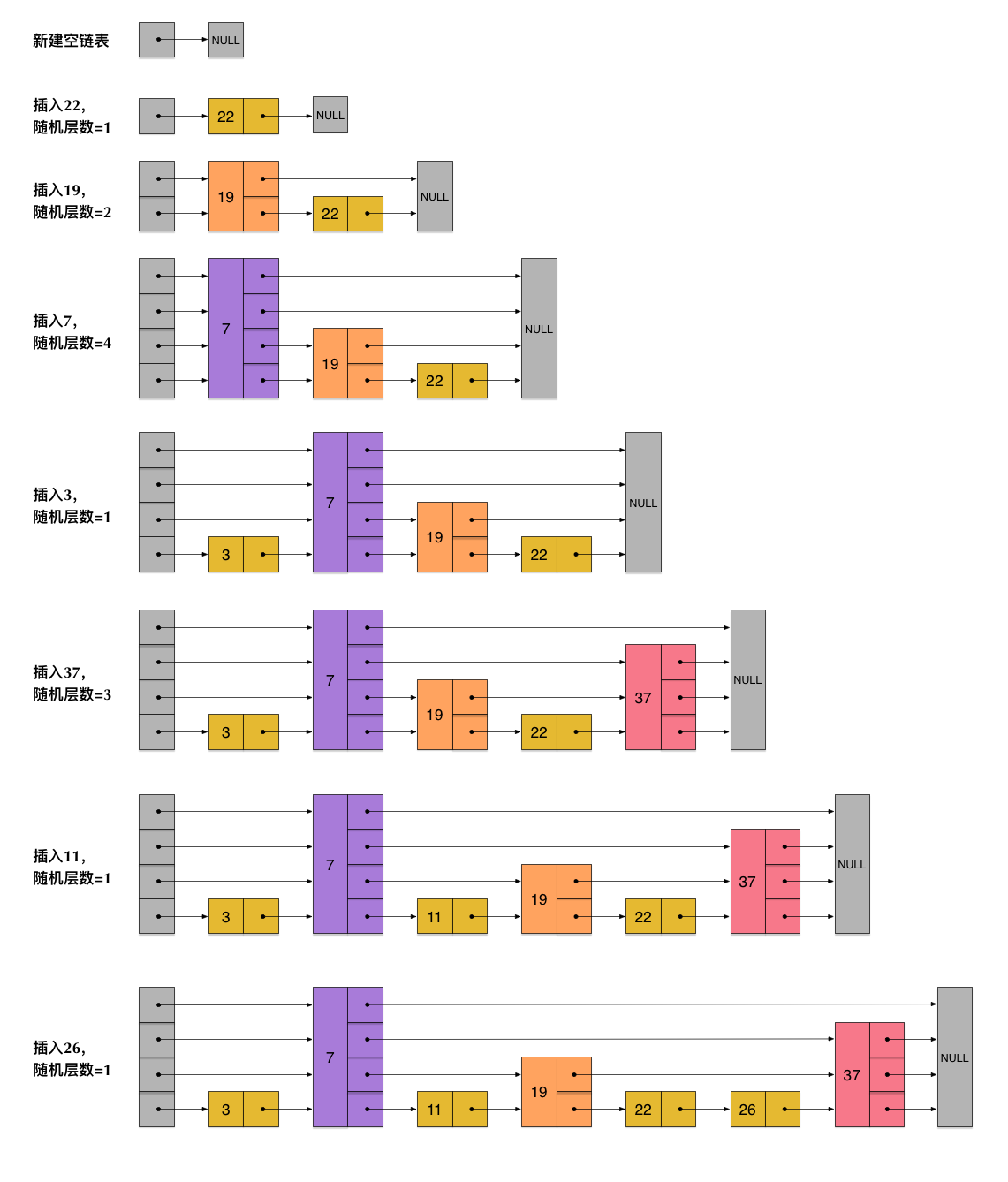``````// Marswiz @2022
// Skiplist in JS.
class SkipList {
MAX_LAYER = 32;
P = 1 / 4;
constructor(data = []) {
this.tail = new Node(Infinity, new Array(this.MAX_LAYER).fill(0).map(i => null));
this.head = new Node(-Infinity, new Array(this.MAX_LAYER).fill(0).map(i => this.tail));
for (let i of data) {
}
}
let p = this.P;
let nextLayer = [null];
while (nextLayer.length < this.MAX_LAYER && Math.random() <= p) nextLayer.push(null);
let curNode = new Node(val, nextLayer);
let curLayer = this.MAX_LAYER - 1;
while (curLayer >= 0) {
while (this.getNext(cur, curLayer).val <= val) {
cur = this.getNext(cur, curLayer);
}
if (curLayer < nextLayer.length) {
curNode.next[curLayer] = cur.next[curLayer];
cur.next[curLayer] = curNode;
}
curLayer -= 1;
}
}
getNext(node, layer) {
return node.next[layer];
}
show() {
let res = [];
while (cur !== null) {
res.push(cur.val);
cur = cur.next;
}
console.log(res);
}
}

class Node {
constructor(val = 0, next = [null]) {
this.val = val;
this.next = next;
}
}
``````

# 一些操作

## 字符串操作

• 字母转化为数值：`a.charCodeAt(0) - 97`; (97是字母a的ASCII码)

## 位运算

• 补码
• 正数的补码是它本身；
• 负数的补码，是符号位保持为`1`不变，其他位取反，然后整体再加1。
• `AND OR XOR`都满足交换律和结合律；
• `(a&b)^(a&c) = a&(b^c)`
• `x^0 = x`
• `x^x = 0`
• `>>`是带符号右移，表示移动过程中左侧空出来位置用符号位的值来填充（正数补0，负数补1）；
• `>>>`是无符号右移，表示移动过程中左侧空出来位置，始终用`0`来填充；
• `a & (-a)` 可以找出数字a的最低非0位

## BigInt

• 除了比较数值大小外(`>`/`<`/`>=`/`<=`)，BigInt只能与BigInt类型进行计算；
• BigInt基本可以表示任意长度的整数，但仍有上界，只是非常大；
• BigInt的位运算：
• BigInt只有带符号左右移操作符`<<``>>`
• BigInt在`<<``>>`的时候，不会被视作`32`位整数，而是它本身。

## 数组操作

### 数组中随机取一个元素

``````let randPos = Math.floor( Math.random() * arr.length )
let res = arr[randPos]
``````

### 数组中删除一个元素

``````arr.splice(position, 1)
``````

### 浅拷贝数组

``````// 1.
Array.from(arr);

// 2.
arr.slice();

// 3.
[].concat(arr);
``````

### 数组sort排序

``````// 字符串升序
arr.sort()
// 字符串降序
arr.sort().reverse()
// 数字升序
arr.sort( (a,b) => a-b )
// 数字降序
arr.sort( (a,b) => b-a )
``````

## 判断变量类型

``````// 精确返回变量类型，首字母大写
Object.prototype.toString.call(arg).slice(8,-1)
``````

## 链表操作

### 链表的前序、后序遍历

``````    function iterate(nodeHead){
// 这里写是前序；
// 这里写是后序。
}
}
``````

## 数值操作

### 辗转相除找最大公约数

``````function gcd(num1, num2) {
return num2 === 0 ? num1 : gcd(num2, num1 % num2);
}
``````

### 找两数最小公倍数

``````let LCM = num1 * num2 / gcd(num1,num2);
``````

### 寻找1 ~ n范围内每个数的约数

``````function findDividers(n) {
let res = new Array(n+1).fill(0).map(i => new Array(0));
for (let i=1; i<=n; i++) {
for (let j=i; j<=n; j+=i){
res[j].push(i);
}
}
}
``````

### ABC集合的合集元素数目

`|A∪B∪C| = |A| + |B| + |C| - |A∩B| - |A∩C| - |B∩C| + |A∩B∩C|`

### 合并区间

1. 先按起始位置`l1,l2,l2.3...`对区间进行升序排序；
2. 声明一个left和一个right变量，用来保存当前区间的左右边界值，以及一个数组res用来保存结果；
3. 从左到右遍历区间数组（索引index从0到n-1），对每个区间`[l1,r1]`和它下一个区间`[l2,r2]`，因为排过序可知此时存在`l2 >= l1`
• 如果l2 > r1，说明下一个区间和现在的区间不重合，直接把现在的结果区间`[left,right]`放入结果数组，并更新left = l2, right = r2；
• 否则l2 <= r1，说明区间存在重合：
• 此时如果r2 <= r1，说明`[l2,r2]`区间被完全覆盖，此时直接跳过；
• 如果r2 > r1，此时更新新的区间右边界值right = r2；

### 最大值 <= M 的子数组个数

1. 每一个子数组都有一个结尾，我们可以通过枚举子数组结尾元素位置，来计数；
2. 对于以`arr[i]`为结尾元素的一系列子数组：
1. 如果`arr[i]`本身`> M`，则没有满足要求的子数组；
2. 如果`arr[i]`本身`<= M`，则以`arr[i]`为结尾的满足要求的子数组个数，等于`i`位置向前(包含`i`)满足`<=M`的连续元素个数.
``````function cal(arr, M) {
let res = 0;
let cur = 0;
for (let i of arr) {
cur = i > M ? 0 : cur+1;
res += cur;
}
return res;
}

// cal([1,5,2,3,4],3);
// 4
``````

### 矩阵旋转

1. 如果旋转90度，可以转化为一次沿水平、垂直的翻转+一次沿对角线的翻转
2. 如果旋转180度，可以转化为两次沿水平、垂直的翻转

## 消除相同的数问题

### 消除相同的两个数

• 如果两个数 `a === b`，那么`a ^ b === 0`
• 任何一个数`a`，它与0异或运算后，仍然是它本身，`a ^ 0 === a`

### 消除相同的多个数

1. 按位思考，对于每一个数的每一位，因为只能是1或0，对于重复出现的n个数，它们在这一位上的加和只能是`n``0`
2. 所以对于除单独的数`x`外，其他的数在每一位上的加和只能是`nk` (k >= 0 && k <= n)；
3. 考虑单独的数x，全部数在每一位上，加起来的和只能是`nk+1``nk`
4. 因此，对全部数每一位进行加和，然后用`n`取余数，余数就是要找的单独数x在该位上的实际值。
``````// 在一个数组 nums 中除一个数字只出现一次之外，其他数字都出现了三次。请找出那个只出现一次的数字。
var singleNumber = function(nums) {
let res = 0;
let cur = 1;
for (let i=0; i<31; i++) {
let s = 0;
for (let n of nums) {
if ((n & cur) !== 0) {
s += 1;
}
}
res += cur * (s % 3);
cur <<= 1;
}
return res;
};
``````

## 位运算判断纯字母字符串是否含有重复字母

318. 最大单词长度乘积

1. `let cur = 1 << i`;
2. `mask |= cur`;

• 如果为0，则没有重复字母；
• 否则含有重复字母。

## 取余操作

### 对较大的幂值进行取余操作

`a ** b % mod`，假设 `a ** b` 是一个较大的值，超出了JS数字的表示范围。

``````let res = 1;
for (let i=0; i<b; i++) {
res *= a;
res %= mod;
}
``````

## 概率问题

### 按照权重随机选取元素

1. 要随机选取，一定要用到随机数API`Math.random()`，但是它只能在一个区间内均匀选取；
2. 要按照权重选取，我们需要将这些权重转化为区间长度，这样在区间内均匀随机选取一个位置，判断其在哪一个区间内，就可以实现按权重选取一个元素了；
3. 具体地：
1. `0`开始，我们将`w[i]`转化为区间长度，则`i`位置对应的区间右边界为`[0,i]`区间内`w[i]`的前缀和，进行预计算，`w[i]`前缀和组成的数组假设为`pre[i]`
2. `w[i]`的总和为`s`，则我们在`[0,s]`范围内随机均匀抽样，选取一个随机数`t`
3. 二分查找，找到第一个`pre[i] >= t`的位置`i`，则`i`是我们按权重随机选择的结果。

### 使用rand7生成rand10

1. 可以多次执行提供的随机函数，生成更多的随机结果；
2. 为保证结果是均匀的，我们不能让两次随机产生的结果互相有叠加，因为这样会让概率值发生变化；

1. 调用两次`rand7()`，分别当做横坐标`r`和纵坐标`c`
2. `r``c`一共可以表示`7*7 = 49`个二维格子位置，将格子从上到下、从左到右进行编号`1 ~ 49`
3. 计算出抽到的格子编号`idx`
4. 因为`49`无法整除`10`，对于后面的`[41, 49]`，如果抽到，我们拒绝采样，直接重新再采样一次，直到抽到的数`idx <= 40`
5. 返回`（idx - 1) % 10 + 1`。（为了让取模后的起始点为`1`而不是零）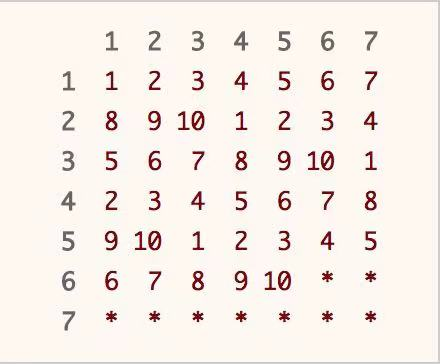# 小技巧： Tips

1. 集合中选取元素组成目标和`tar`的问题，可以转化为给集合中的元素添加符号的问题：
• 抽取若干元素凑成目标和：转化为在原集合中的每个元素前面添加`+1``0`因子；
• 将集合中的元素，分为各自加和为目标和的两组：转化为在原集合中的每个元素前面添加`+1``-1`因子，使得加和结果为`0`
• 选取集合中的部分元素，分为各自加和相同的两组：转化为在原集合中的每个元素前面添加`+1``-1``0`因子，使得加和结果为`0`
2. 判断数组的前`n`个元素`arr ~ arr[n-1]`是否与索引值一一对应（也就是元素取值范围是`[0, n-1]`）：
• 从前向后遍历原数组，记录最大值`max`，如果`max === i`，则`[0,i]`区间满足索引与元素一一对应。